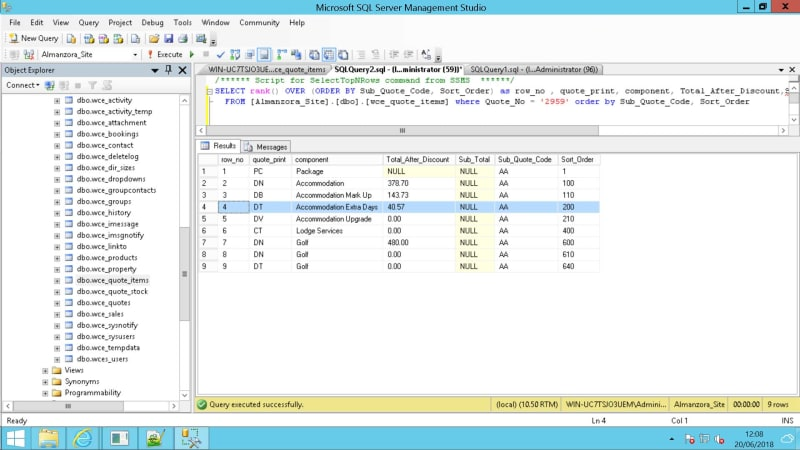####Sum Subquery

Hi There,

I hit an issue today that I am scratching my head with, any guidance would be much appreciated.

I am trying to come up with a query that SUMS all the rows 'Total_After_Discount' field values above and including when the row where the value in the quote_print = 'DT is found and for the sub_total field on the 'DT' row to be populated with the value of the lines above including the DT line. So in the example, row_no 1,2,3,4 add the Total_After_Discount values for these rows together and set line 4's subtotal with the value, in this example 563.

Then from row_no 5 to the next rows where the quote_print value = 'DT' row_no 9 in the example do the same. So the sub_total field for row_no 9 would be set to 480.

So every time an instance of quote_print = 'DT' stop add this line and all lines above's Total_After_Discount values and set the DT row sub_total with the calculation.

I hope that makes sense!

I have this example query that returns the following (see screenshot). Thanks for looking and any help you provide.

Code:
```SELECT rank() OVER (ORDER BY Sub_Quote_Code, Sort_Order) as row_no , quote_print, component, Total_After_Discount, Sub_Total, Sub_Quote_Code, Sort_Order
FROM [Almanzora_Site].[dbo].[wce_quote_items] where Quote_No = '2959' order by Sub_Quote_Code, Sort_Order```